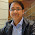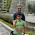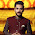## Friday, December 23, 2011

### Multiple level sort using VBA

If you want to sort data on multiple levels in a range .Snapshot below-

Here is the code-

Sub MULTI_LEVEL_SORT()
'1ST level SORT ON NAME COL A
'2ND level SORT ON START DATE COL B
'3RD levle SORT ON END DATE COL C
Sheets("Sample").Range("a1:d" & Sheets("Sample").Range("a1").End(xlDown).Row).Sort _
key1:=Sheets("Sample").Range("A:A"), order1:=xlAscending, _
key2:=Sheets("Sample").Range("B:B"), order2:=xlAscending, _
key3:=Sheets("Sample").Range("C:C"), order3:=xlAscending, _
End Sub

1.If you are interested in learning Multiple Level Sorting Using VBA

http://www.exceltip.com/tips/multiple-level-sorting-using-vbain-microsoft-excel-2010.html

2.This macro is perfect. Thank you very much. I used it in a macro from Cols A to W and for a sheet called "Misc". It works perfectly.

1.Hi,
Would you please share your code of multi-level sorting as for me till 3rd level it is working fine but from 4th its started failing.

2.3.